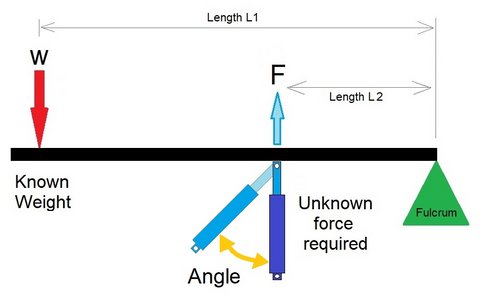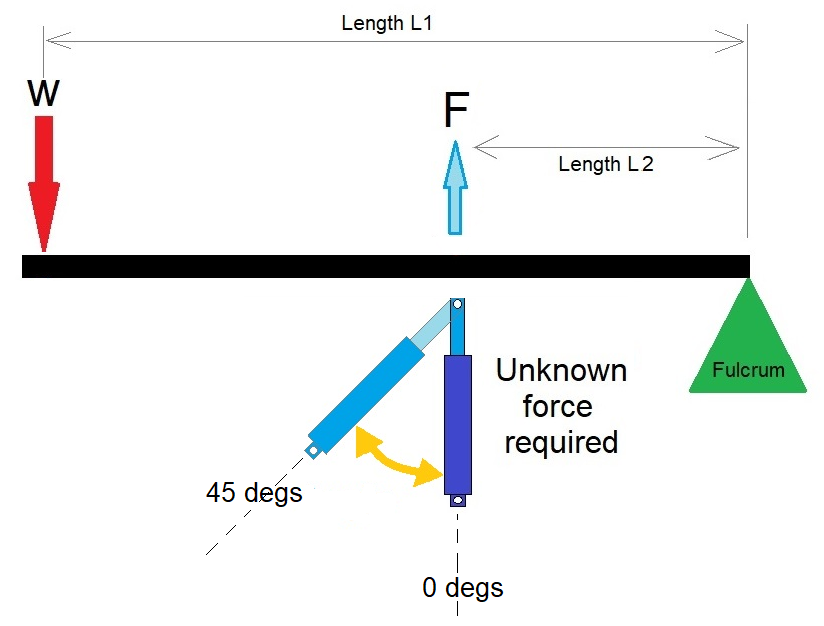# 3rd Class lever with the force on an angle

## How to calculate a 3rd Class lever where the pushing or pulling force is on an angleWe have previously written about  3rd class levers in another article, and created an online calculator for them, (Link below).

3rd Class lever article and Calculator

However in this article we help you understand what happens to the force requirements when the pushing or pulling force applied, is placed on an angle.

When a force is applied at an angle to the lever arm in a second-class lever, it can be split into two components: a perpendicular component and a parallel component relative to the lever arm. The perpendicular component of the force is responsible for creating the torque that moves the load, while the parallel component will push the lever arm sideways.

To calculate the force required to move the load in a third-class lever when the force is applied at an angle, trigonometry is used to determine the magnitude of the perpendicular component of the force.### How to calculate the force required?

To calculate the force required for a third-class lever when the force required is pushing on an angle, you can use the following formula:

F = (w1 * L1) / (L2 * sin(theta) + L1 * sin(phi))

where:

• F is the force required to move the load, in newtons
• w1 is the weight of the load, in newtons
• L1 is the distance from the fulcrum to the force, in meters
• L2 is the distance from the fulcrum to the load, in meters
• theta is the angle between the lever arm and the force, in radians
• phi is the angle between the lever arm and the load, in radians

The formula for calculating the force required for a third-class lever when the force required is pushing on an angle is similar to the formula for a second-class lever, except that an additional term is added to account for the angle between the lever arm and the load.

 Length from Actuator to Fulcrum (L2): mm cm m inches feet Length from Load to Fulcrum (L1): mm cm m inches feet Angle of Force: degrees Weight of Load (w1): g kg lbs N Force Required:### What if the lever is also on an angle, how does this affect the calculation?

If the lever is also on an angle in the third-class lever system, the calculation for the force required to move the load will become more complex, as it will involve multiple angles.

In general, if the lever arm is also on an angle, the perpendicular component of the force applied to the lever arm will be affected by the angle between the lever arm and the force, as well as the angle between the lever arm and the load.

To calculate the force required to move the load in a third-class lever system with both the force and the lever arm on an angle, the same formula can be used:

F = (w1 * L1) / (L2 * sin(theta) + L1 * sin(phi))

However, the angles theta and phi will need to be adjusted to account for the angle of the lever arm.

If the lever arm in a third-class lever system is at a 45-degree angle, the calculation for the force required to move the load will depend on the angles between the lever arm, the force, and the load.

Assuming that the angle between the force and the lever arm is theta, and the angle between the load and the lever arm is phi, the calculation for the force required to move the load in a third-class lever system with a lever arm at a 45-degree angle can be written as:

F = (w1 * L1) / (L2 * sin(theta + 45) + L1 * sin(phi - 45))

Here, the angles theta and phi are adjusted by adding or subtracting 45 degrees to account for the 45-degree angle of the lever arm.

To calculate the force required to move the load, you would need to measure or calculate the values for L1, L2, w1, theta, and phi, and plug them into the formula to solve for F.

Please note that this is just an example, and the specific calculation for a given situation may vary depending on the specific angles and measurements involved in the lever system.

### What would be a typical application for a 3rd class lever where the pushing force is on an angle?

A typical application for a third-class lever where the pushing force is on an angle is a human arm.

In the human arm, the elbow joint acts as the fulcrum, and the biceps muscle attaches to the forearm bone (load) and pulls on it to generate a lifting force. However, the biceps muscle is located on the front side of the arm, while the load is located on the back side of the arm. This means that the biceps muscle exerts a force on the arm at an angle, which creates a third-class lever system.

When the biceps muscle contracts, it exerts a force on the forearm bone at an angle, causing the forearm to move upward. The perpendicular component of the force generated by the biceps muscle provides the torque that moves the forearm bone, while the parallel component of the force simply pushes the bone sideways.

By using a third-class lever system with the pushing force on an angle, the human arm is able to move loads with more speed and range of motion, although the trade-off is that more force is required to lift the same weight compared to a second-class lever system.

### What are the advantages for a 3rd class lever where the pushing force is on an angle?

The advantages of using a third-class lever where the pushing force is on an angle include:

1. Increased range of motion: By using a third-class lever with the pushing force on an angle, it is possible to increase the range of motion and precision of movement. This is because the lever arm can be positioned at different angles, which allows for more control over the direction of the force and the movement of the load.
2. Speed: The third-class lever with the pushing force on an angle can allow for fast movements of the load, which can be beneficial in situations where speed is important. This is because the force required to move the load can be applied with a quick and precise movement of the lever arm.
3. Mechanical Advantage: Although third-class levers with the force on an angle require more force to move a load compared to second-class levers, they can still provide a mechanical advantage. The lever arm can be positioned at different angles, which can help to increase the torque and make it easier to move the load with less force.
4. Improved Control: The angle of the force applied in a third-class lever with the force on an angle allows for improved control over the movement of the load. This can be useful in situations where the load needs to be moved around obstacles or in tight spaces.

Overall, using a third-class lever with the force on an angle can provide increased range of motion, speed, and precision, while still providing a mechanical advantage and improved control over the movement of the load.